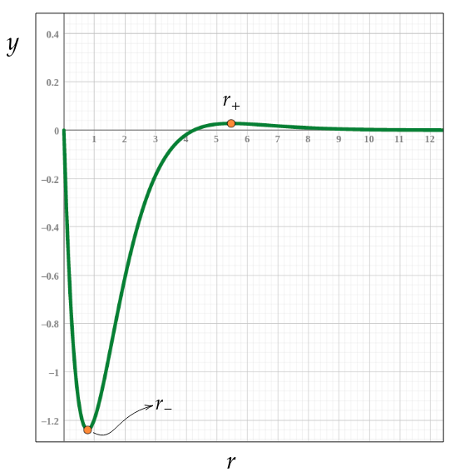# Consider the following function of variable r, for r > 0: y(r) = (r^2 - 4.25r) exp(-r). a. Find...

## Question:

Consider the following function of variable r, for {eq}r > 0: y(r) = (r^2 - 4.25r) exp(-r) {/eq}.

a. Find the value of r where y takes its minimum value in the {eq}r \geq 0 {/eq} interval.

b. Find the value of r where y takes its maximum value in the {eq}r \geq 0 {/eq} interval.

## Finding the extrema of a function

The extrema are the maximum and minimum values of a function. When we search for maximum and minimum of a given function {eq}f( r) {/eq}, we are in fact looking for the points where the inclination of the tangent line is zero. Since the derivative gives the inclination of the tangent line at a given point, the maximum and minimum values can be obtained by application of the condition

$$\dfrac{\mathrm{d} f}{\mathrm{d} r} =0 \ .$$

Hence, the solution of the equation above gives all the maxima and minima of the function.

We have to find the extrema of the function

$$y( r) =\left( r^{2} -4.25r\right)\exp( -r) \ .$$

The derivative of this function gives

\begin{align*} \dfrac{\mathrm{d} y}{\mathrm{d} r} & =\left[\dfrac{\mathrm{d}}{\mathrm{d} r}\left( r^{2} -4.25r\right)\right]\exp( -r) +\left( r^{2} -4.25r\right)\dfrac{\mathrm{d}}{\mathrm{d} r}\exp( -r)\\ \\ & =( 2r-4.25)\exp( -r) +\left( r^{2} -4.25r\right)( -1)\exp( -r)\\ \\ & =-\ \left( r^{2} -6.25r+4.25\right)\exp( -r) \ . \end{align*}

Now we need to impose

$$\dfrac{\mathrm{d} y}{\mathrm{d} r} =0\ ,$$

and since the exponential function is anywhere different from zero except on the special limit of {eq}r \rightarrow \infty {/eq}, this implies

$$r^{2} -6.25r+4.25=0\ .$$

This is a quadratic equation whose solutions are given by

$$r=\dfrac{6.25\pm \sqrt{( -6.25)^{2} -4( 1)( 4.25)}}{2} \ .$$

The solutions are

$$r_{+} =5.47\ ,$$

and

$$r_{-} =0.776\ .$$

Conclusion

a. The value of {eq}r {/eq} where {eq}y {/eq} takes its minimum value is {eq}r=0.776 \ {/eq}.

b. The value of {eq}r {/eq} where {eq}y {/eq} takes its maximum value is {eq}r=5.47 \ {/eq}.

Below is a graph of {eq}y {/eq} in the range {eq}r\geqslant 0 {/eq}.